# How to solve fermi estimation

tamagoyaki1999.hatenablog.com

Consultants have a unique method of thinking. So, I recommend you to read some books about X-thinking.

In the case interview, there are 3 types of question.

1. fermi estimation
2. recommendation of strategy
3. chat

Fermi estimation is a thinking game about unkown situation, such as market size of 〜〜.
Recommendation strategy is a role play game of consulting.
In many cases, you anser for fermi estimation, then recommend the strategy like how to maximize the revenue.

### Fermi estimation

In the fermi estimation game, you make a equation and estimate the value.
To solve this question, all you have to do is to master one equation shown below.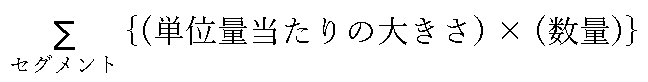Sigma could be a good appeal to the interviewer who is unfamiliar with mathematics.
When you try a Fermi estimation, I recommend you to write this equation.
If you practice to use this equation every time, you can pass interviews of consulting farms easily.
Next, I'll show how to use this equation.

### size per unit quantity × quantity

revenue ＝ unit price × quantity
This equation is very popular. As you know, every number can be factored like this.
The examples are shown below.

• distance ＝velociry × time

velocity ＝ distance per unit time

• area of rectangle ＝ width ×height

width ＝ area per unit height

These are basic equations. And you can create original equaton. For example,

velocity ＝ velocity per unit weight × weight

To solve fermi estimation, it is important to create equatons which make your thought easier.

### unit thinking

When you create new equation, you must care dimension. It is famous for engineer.

To think revenue by dimension,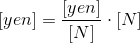To think weight by dimension,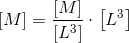in this case, weight is a function of density and volume,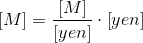in this case, weight is a function of weight per unit cost and cost. It is like selling by weight in a supermarket.
You should check if the left side and right side of the equation are match.

#### procedure of solving fermi estimation

1. Get an agreement on the dimension of the answer

When the question is about market size, the dimension of the answer is yen (dollar). When profit, the dimension is % (yen/yen). To answer properly, you should get an agreement on the dimension.

1. Set up an equation

To set up an equation, numbers that you already know, for example population, number of days in a year, life expectancy, land area of ​​Japan, are screened at first. Then, you divide the dimension that you got agreement by the dimension of the number.
If you can guess revenue per day, you can set up an equation below,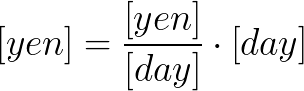Then you put numbers into the formula.

#### How to study fermi estimation

Let's factor numbers around you!

##### Exercise
• Distance from Tokyo to Fukuoka
• Weight of a case of beer
• the number of passengers ride on Yamanote line in a day
• Thickness of a tissue
• The number of diapers which a baby uses per day

### Segmentation

As quantity per unit and quantity depend on the case, it is impossible to determine the number.
In that situation, you can divide cases, then sum them.

When you think the number of bottles of beer consumed in a year, how do you think?
Guessing the number of bottles per person, then multiply the population?
You soon find that minors can't buy beer, women who have babys sometimes refrain from beer and so on. Because of this, the accuracy of estimstion become bad.
In that cases, it is successful to divide cases.

#### The method of dividing by popular index

In the case interview, it is effective to divide by popular index.
Pros. :

• Easy to understand

Cons. :

• Old thought
• No surprising
• Somtimes no reality

The examples of popular index are population, area of country, life expectation, age, sex, to C or to B.
People who work in the media use the words like F1 which is a category name divide people by age and sex.
It is sometimes recognized old-fashion but it is very effective for the case interiew.
Of course diversification is progressing, nevertheless the life style of people who are same age is same trend.
It is difficult for the interviewer to disagree this trend.

#### the method of classification by use-case

It is the method to create ideas of index from use-cases as the case of beer written before.
Pros. : Because you have the reason why you select the index, you can explain your idea logically, appeal your ability to find points of discussion to the interviewer, handle a difficult question and make use of it for a proposal.
Cons. : It takes longer time. Your idea can be subjective.

#### Exercise: To estimate the market size of drone.

You can classify the segments by age and sex. But it is very difficult to find the evidence of drone ownership. Then all you can do is to assume the ownership of each generation intuitively then multiply them by population. It is a boring idea.
In thus case, classification by use-case is useful.

What is drone used for?
Derivering, taking a picture from sky, toys, sports gear.
Dividing by toC toB seems helpful.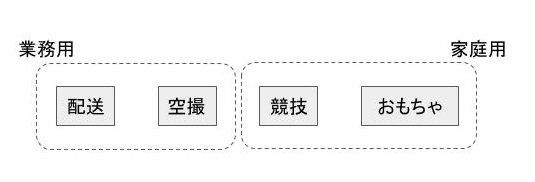What kind of function should the drones have?
Bringing, grabbing, wathing, flying, controlling.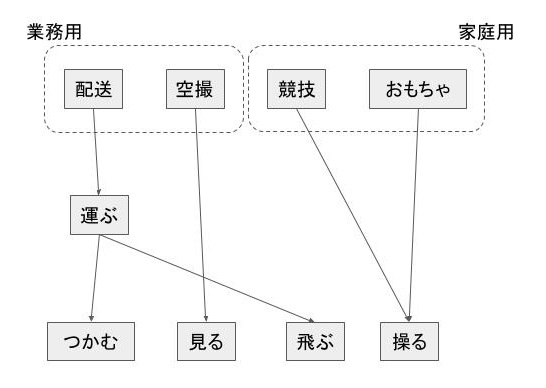What does realize the functions?
Robot arms, sensor, jet, micon.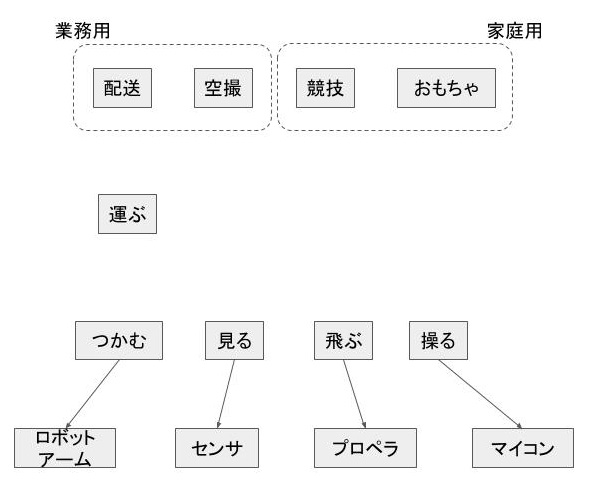Seeing this figure, you may find applications like construction drone.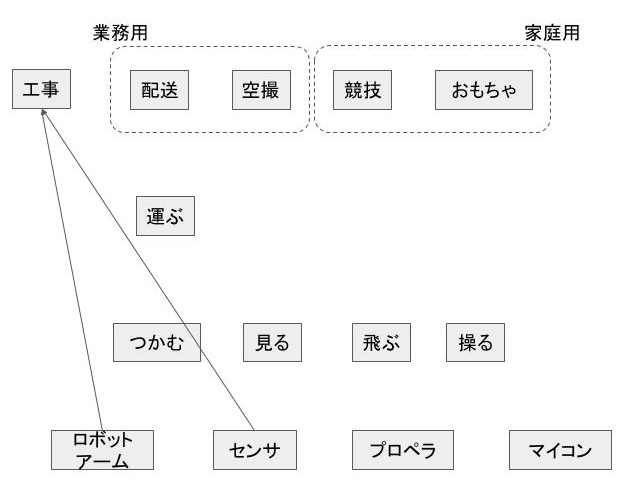You can get many ideas. This method makes you to consider comprehensively.
Here, the method of abstruction up and down is used. This method is useful for creating ideas.